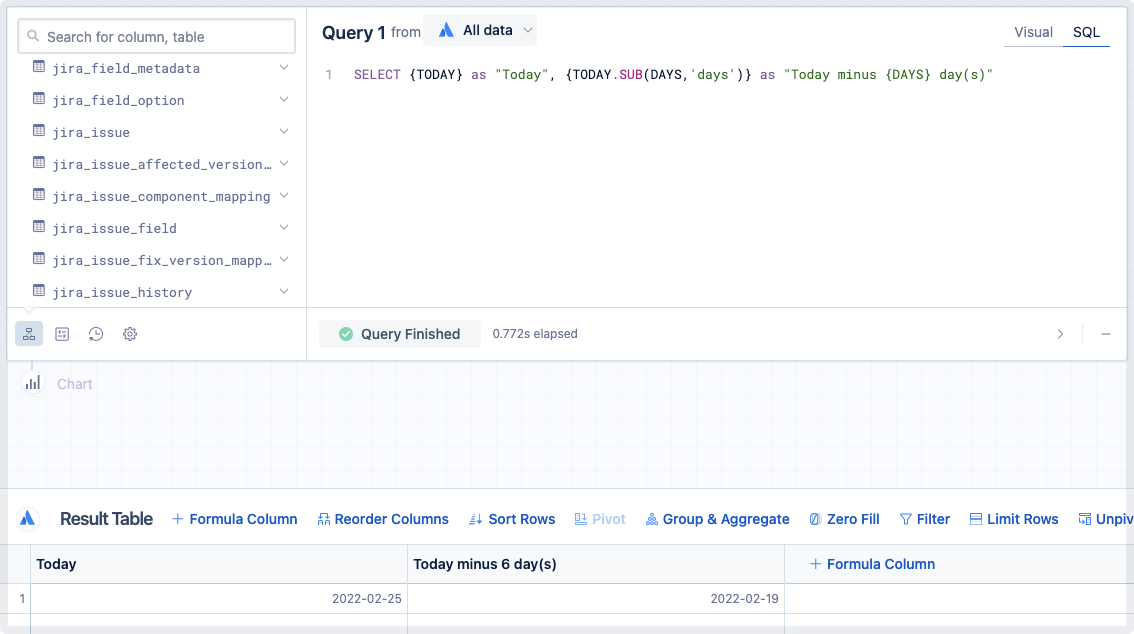# Date and time functions for variables

You can perform functions on relative date variables and datetime dashboard variables to customize the date returned to fit your needs. Learn more about variables.

The following date and time functions are available:

• SUB()

• PART()

• BUCKET()

Try it out for yourself with this quick example:

1. Select Create > Chart from the global menu.

2. Switch the query to SQL mode.

3. Enter the following statement into the SQL editor: SELECT {CURRENT_CALENDAR_YEAR.START.SUB(1,'day')}

This returns the last day of the previous year.

## Units of time

You can use the following units of time in any of the date and time functions:

• day

• week

• month

• year

• quarter (can use only in the PART function)

You can use either the singular or plural form of these units.

You can add or subtract units of time from datetime variables to calculate other dates.

```1 ````{DATE_VARIABLE.ADD(integer, 'units')}`

To subtract from datetime variables, use the SUB function:

```1 ````{DATE_VARIABLE.SUB(integer, 'units')}`

Make sure to replace:

• DATE_VARIABLE with the datetime variable you want to modify

• integer with the number of units you want to add or subtract from the variable

• units with one of the available unit options; note that the units are wrapped in single quotes

### Examples using the SUB function

#### Get the first day of the previous month

You can use the SUB function to subtract one month from the start date of the current month:

```1 ````{CURRENT_MONTH.START.SUB(1,'month')}`

#### Get the last day of the previous month

You can use the SUB function to subtract one day from the start date of the current month:

```1 ````{CURRENT_MONTH.START.SUB(1,'day')}`

## PART function

PART() lets you extract specific parts of a date and returns it as a number, which you can use in other calculations.

Here’s the general format:

```1 ````{DATE_VARIABLE.PART('units')}`

Make sure to replace:

• DATE_VARIABLE with the datetime variable you want to extract from

• units with one of the available unit options; note that the units are wrapped in single quotes

### Examples using the PART function

#### Get the quarter of the current day

```1 ````{TODAY.PART('quarter')}`

If today was March 30, 2020, this would return 1.

#### Get the last day of the current month

```1 ````{CURRENT_MONTH.END.PART('day')}`

If the current month was March, this would return 31.

#### Get the current year

```1 ````{TODAY.PART('year')}`

If today was March 30, 2020, this would return 2020.

## BUCKET function

BUCKET() lets you convert a date into the specified time bucket and returns it as a date string.

Here’s the general format:

```1 ````{DATE_VARIABLE.BUCKET('units')}`

### Examples using the BUCKET function

#### Get the month and year of the previous month

```1 ````{CURRENT_MONTH.START.SUB(1, 'month').BUCKET('month')}`

If the current month was March 2020, this would return 2020-02.

This is also a great example of how you can chain together the SUB function and BUCKET function!

#### Get the ISO week date of the current day

```1 ````{TODAY.BUCKET('week')}`

If today was March 30, 2020, this would return 2020-W14.

#### Get the quarter date of the current day

```1 ````{TDOAY.BUCKET('quarter')}`

If today was March 30, 2020, this would return 2020-Q1.

## Combine date and time functions

Relative date variables are useful when looking to filter your data on flexible date ranges. Sometimes, applying a single date and time function may not be as flexible as you need. In those cases, you can chain together multiple date and time functions and apply them to your variables.

For example, what if you wanted to get data for one full week up to yesterday? You can combine these two relative date variables, {TODAY.SUB(1,'day')} and {TODAY.SUB(1,'week')}, into the following variable as your start date:

```1 ````{TODAY.SUB(1,'day').SUB(1,'week')}`

You can then use the following as the end date to get the day before today (in other words, yesterday):

```1 ````{TODAY.SUB(1,'day')}`

If today was March 30, 2020, these would return 2020-03-22 and 2020-03-29, respectively.

## Dashboard variables nested in date and time functions

If you need to reference a “Hidden variable”, “Text input”, or another type of control within a date and time function, you’ll need to omit the curly braces around its dashboard variable.

For example, let’s say we have a “Text Input” control called DAYS that takes in integer values. We want to use the its value in a date and time function so we can dynamically select data created # days before today, where # is the value of the {DAYS} variable. Our function would look like this:

```1 ````{TODAY.SUB(DAYS, 'days')}`